## You need to test, we're here to help.

You need to test, we're here to help.

## 09 May 2022

### Signal and Power Integrity Tutorial: Measuring Clock Jitter Sensitivity to Power Rail Noise, Pt. 2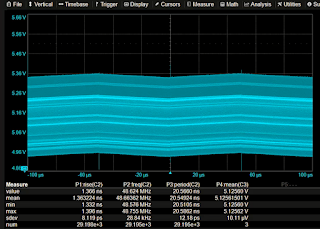Figure 1. 400 mVpp oscillation on the power traceis due to 48 MHz clock noise.

In Part 1, we used a function generator to create a power source with a known perturbation. Seeing that the noise on the power rail and the clock period were synchronous when we observed both traces together using a WavePro HD oscilloscope, we knew that there was a clear relationship between the two to be further investigated. Now, we're ready to examine more closely how the clock jitter responds to voltage variations on the power rail.

### 5. Eliminate the Power Trace Oscillation with Filters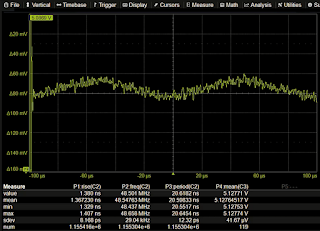Figure 2. Low-pass filter on the power rail signal removes the high-frequency oscillation and allows observation of the 10 kHz perturbing signal.
However, at this stage, we can’t accurately measure the voltage variation amplitude on our power trace because of the clock noise causing a 400 mVpp oscillation. This clock noise is swamping the 10 kHz perturbation signal and needs to be eliminated before we can measure the voltage variation amplitude.

The simplest way to accomplish this is to filter the power trace and conduct our measurements on the filtered signal. The interfering noise has a 48 MHz frequency, the perturbation frequency is 10 kHz, so a low-pass filter with a cutoff frequency of about 1 MHz should remove the 48 MHz oscillation. Figure 2 shows the effects of applying a 4th order Butterworth low-pass filter with a cutoff frequency of 1 MHz to power trace C3. Now, we can observe the effects of the 10 kHz perturbing signal on the power trace.

### 6. Situational Awareness: Understand Effect of Circuit Design on Power Trace

Before we connected the power source to the clock, our example power trace was a 10 kHz square wave with a 200 mV peak-to-peak amplitude. Now, it appears to be a triangle wave of 10 kHz, but with an amplitude of about 20 mV. What happened?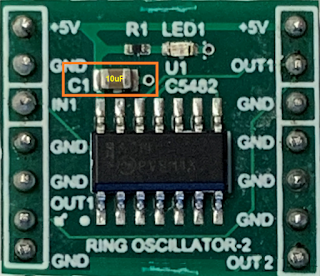Figure 3. 10 µF bypass capacitor between the 5 Vpower rail and ground forms a low-pass cutoff filter.
The answer can be found by observing the design of our circuit board. There is a 10 µF bypass capacitor between the 5 V power rail and ground, shown in Figure 3.

The 10 µF capacitor loading the 50 Ω power source forms a low-pass filter with a cutoff frequency of 318 Hz.  The low-pass filter is an integrator. The RC combination has a time constant of 500 µs, so the 10 kHz waveform has a positive half cycle period of less than one tenth of the time constant. No wonder it only rises to 20 mV during the positive half cycle of the square wave. Nevertheless, this waveform, as it is, is adequate to calculate the clock jitter sensitivity to power rail voltage variations, because it has a measurable variation in the power rail voltage and a related jitter response, which are key to making our calculation.

### 7. Calculate the Clock Jitter Sensitivity to Rail Voltage

Now, we turn on the track of our clock period standard deviation, which for our purposes, is the clock jitter track. With the track vertical scale at 50 ps/div, we move it onto the same grid as the power trace, so that they overlay one another (Figure 4). We increase the amplitude of the power source (function generator output) until the perturbing signal amplitude on the power trace is about 4 divisions (80 mV).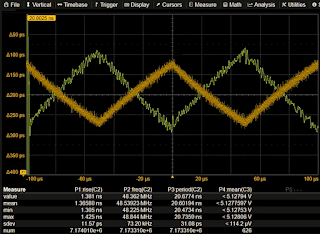Figure 4. The voltage variations on the power rail shown in the same grid as the clock period track (jitter track). These variations form the basis of the clock jitter sensitivity measurement.
When we compare the traces, it is obvious that they are related. Both are synchronous with the trigger on the power source. The clock jitter track is inverse to the power rail voltage variation because the propagation delay of the inverters in the ring oscillator decreases as the power rail voltage increases, raising the oscillator frequency and lowering its period.

The sensitivity of the clock period jitter to the voltage variations on the power rail can be calculated by taking the ratio of their peak-to-peak amplitudes.

In our example, the clock jitter variation is about 150 ps while that of the power rail voltage is about 80 mv. Taking their ratio, the clock jitter sensitivity is about 1.85 ps/mV, rounded up to 2 ps/mV to keep calculations simple.

Now that we know the clock jitter sensitivity to variations of the power rail voltage, we can anticipate the clock period jitter if we know the voltage variation. Likewise, if we measure the clock jitter, we can anticipate the voltage variation that produced it.

### 8. Verify Clock Jitter Sensitivity to Rail Voltage Variations

This measured sensitivity to voltage variations can be verified by repeating the measurement using a different power source. When we exchange the power supply connected to the clock to a switched-mode power supply, we get a different instance of power rail voltage variations and related clock jitter, shown in Figure 5.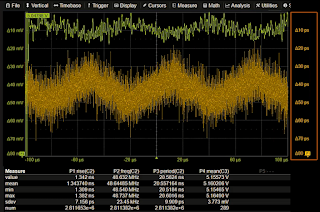Figure 5. Switched-mode power supply (top) showspredictable 10 mV voltage variation, given theestimated 20 ps amplitude of the clock jitter trackand the calculated sensitivity of 2 ps/mV. The jitterscaling has been appended at right for convenience.
This power supply (upper trace) has voltage variations of about the same 10 kHz frequency as did our function generator. In this case, its due to the switching frequency. The estimated amplitude of the clock jitter track (lower trace), ignoring the random noise, is about 20 ps. Given the clock jitter sensitivity we calculated at about 2 ps/mV, we can estimate the power rail voltage variation should be about 10 mV. Looking at the power rail voltage, again ignoring the random noise, we see that the amplitude is indeed about 10 mV.

By tracking the clock period measurement to obtain the pattern, or the “signature”, of the jitter variation, we can compare that to the fingerprint of other features in our circuit, in this case, the power rail voltage. Taking the ratio of the peak-to-peak amplitudes of the jitter track and the underlying modulation of the power rail, we can calculate the clock jitter sensitivity to voltage variation. Switching from a clean to two different perturbed power sources in the process acts as a consistency check of our findings.

Want to try this with your clock source? Download our step-by-step tutorial, Measuring Clock Jitter Sensitivity to Power Rail Noise.

You can also watch Dr. Eric Bogatin demonstrate in the on-demand webinar, “The Impact of Power Rail Noise on Clock Jitter.”